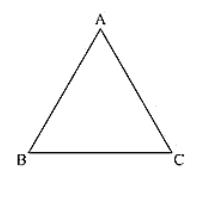# Prove that each angle of an equilateral triangle is 60°.

Question:

Prove that each angle of an equilateral triangle is 60°.

Solution:

Given to prove each angle of an equilateral triangle is 60°.

Let us consider an equilateral triangle ABC.

Such that AB = BC = CA

Now, AB = BC

∠A = ∠C ... (i) [Opposite angles to equal sides are equal]

And BC = AC

∠B = ∠A ... (ii)

From (i) and (ii), we get

∠A = ∠B = ∠C  ... (iii)

We know that

Sum of angles in a triangle = 180

∠A + ∠B + ∠C = 180

∠A + ∠A + ∠A = 180

3∠A = 180

∠A = 60

∠A = ∠B = ∠C = 60

Hence, each angle of an equilateral triangle is 60°.